Mechanics

# Average speed

Average velocity is a quantity that indicates the displacement of a body in a given time.

The average velocity is a quantity characterized by the variation of the position of a body in a given time. Based on dividing the space traveled by the time it took to travel, it is possible to find the velocity of the body: V m = ΔS/Δt. The most common unit of measurement is km/h, however, in the International System of Units ( SI ) , the unit of speed is m/s.

## Average speed summary

• Average velocity is a quantity characterized by the displacement of a body in a given time.
• The most used measurement units for speed are: km/hem/s.
• The scalar velocity is considered only the module, without thinking about the direction or sense.
• The average velocity is a vector quantity, so it has: magnitude, direction and sense.
• The displacement is defined by the end position minus the initial position of the body.

## What is average speed?

The average velocity is given by dividing the displacement of a body in a given time interval . Unlike instantaneous velocity, which only shows us the velocity of the body at a precise point, the average velocity does not take into account the acceleration during the journey. In this way, only the starting point and the arrival point are considered, regardless of whether the body took breaks, increased or decreased speed along the way.

## average speed formula

For the average velocity, it is necessary to know the displacement of the body and the displacement time: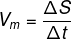• m : average speed (m/s);
• ΔS: displacement (m);
• Δt: time interval (s).

The unit of measurement for speed in the International System of Units (SI) is given in meters per second (m/s) , but the kilometer per hour (km/h) is often used. Thus, it is possible to convert the values ​​directly from km/h to m/s, and vice versa, as follows:

As 1 km is equivalent to 1000 meters and 1 hour is equivalent to 3600 seconds, we arrive at this value of 3.6. So, to transform a value from km/h to m/s , just divide it by 3.6, and to transform a speed from m/s to km/h, just multiply it by 3.6.

## How to calculate average speed

In some cases, where displacement and time values ​​are not given, it is possible to use the velocity equation as follows:

To find the offset:

ΔS = V m .Δt

For the time: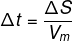In addition, it is possible to find the average velocity through the tangent line of a graph of position as a function of time:

The steeper the line, the higher the average speed. In this situation, the velocity can be called the slope of the line . To find it, just take 2 points on the y-axis and on the x-axis: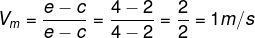Example

A family travels from São Paulo to Rio de Janeiro to spend their holidays. The trip lasts 6 hours on a route of 435 km. What is the average speed of the family car?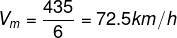## The average speed and movement

Depending on the type of movement involved in the exercise, the average speed may vary.

• Uniform rectilinear motion (MRU) : in the MU, the average velocity is constant and equal to the instantaneous velocity, that is, the body does not accelerate.
• Uniformly Varied Rectilinear Motion ( MRUV ) : In the MUV, the velocity varies uniformly. In this way, there is acceleration and the average velocity is different from the instantaneous velocity.

# Differences between average speed and average climbing speed

Both the average velocity and the average speed find an average value of velocity during a path in which there was a change in velocity, that is, a path that had acceleration.

The difference between the two quantities is that the average speed is vector , it needs the magnitude (value), the direction and the direction of the movement, while the average speed is a scalar quantity , in which only the information of its value is needed, disregarding direction and meaning.

Thus, when we talk about average velocity, we measure the displacement, which is also a vector quantity, considering the end point and the initial point of the movement. At average speed, we measure the distance traveled, which can never give a null value.

## vector average speed

To calculate the average vector velocity, it is necessary to consider the vector sum . In graphical form, each vector is placed with the end snapped to the beginning of the next vector.

The resulting vector is obtained by joining the beginning of the first vector with the end of the last one. In this way, it is possible to find the velocity vector or the displacement vector.

## Solved exercises on average speed

question 1

(Fuvest) Driving to a nearby city, on a flat highway, a driver estimates his travel time, assuming he can maintain an average speed of 90 km/h. When surprised by the rain, he decides to reduce his average speed to 60 km/h, staying there until the rain stops, fifteen minutes later, when he resumes his initial average speed. This temporary reduction increases your travel time, relative to the initial estimate, by:

a) 5 minutes

b) 7.5 minutes

c) 10 minutes

d) 15 minutes

e) 30 minutes

Resolution:

Alternative A

In the 15 minutes (1 quarter of an hour) when the driver’s average speed was 60 km/h, he traveled:

ΔS = V m .Δt

ΔS = 60.0.25 = 15 km

If he had maintained the 90 km/h, he would have covered that 15 km in:

Δt = 15/90 = 0.16 h = 1/6 h

Seeing this value in minutes:

1 hr → 60 min

1/6 hr → 10 min

So, in relation to the decrease in speed, there was an increase of: 15 – 10 = 5 minutes.

(Unicamp) The complete tour of the Sugarloaf Mountain complex includes a cable car stretch of approximately 540 m, from Praia Vermelha to Morro da Urca, a walk to the second station at Morro da Urca, and a second cable car stretch of approx. 720 m, from Morro da Urca to Sugarloaf Mountain. The average speed of the cable car in the first stretch is V 1 = 10.8 km/h , and in the second it is V 2 = 14.4 km/h. Assuming that, on a certain day, the time spent walking on Morro da Urca plus the waiting time at the stations is 30 minutes, the total time for the complete walk from Praia Vermelha to the Sugarloaf Mountain will be equal to:

a) 33

b) 36

c) 42

d) 50

Resolution:

Alternative B

First, it is necessary to find the travel time of each cable car.

For the first trolley: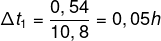For the second cable car: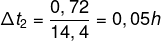This time lasts: 0.05 h + 0.05 h = 0.1 h

Turning it into minutes:

1 hr → 60 min

0.1 h → 6 min

With the wait at the station, the total journey takes: 6 + 30 = 36 minutes